## Arithmetic-Geometric Mean

The arithmetic-geometric mean (AGM)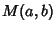of two numbersand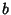is defined by starting with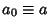and, then iterating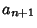(1)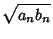(2)

until.andconverge towards each other since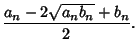(3)

But, so(4)

Now, addto each side(5)

so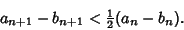(6)

The AGM is very useful in computing the values of complete Elliptic Integrals and can also be used for finding the Inverse Tangent. The special valueis called Gauss's Constant.

The AGM has the properties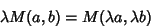(7)(8)(9)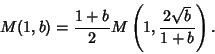(10)

The Legendre form is given by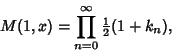(11)

where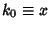and(12)

Solutions to the differential equation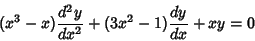(13)

are given by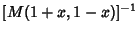and.

A generalization of the Arithmetic-Geometric Mean is(14)

which is related to solutions of the differential equation(15)

When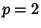or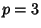, there is a modular transformation for the solutions of (15) that are bounded as. Lettingbe one of these solutions, the transformation takes the form(16)

where(17)(18)

and(19)

The casegives the Arithmetic-Geometric Mean, andgives a cubic relative discussed by Borwein and Borwein (1990, 1991) and Borwein (1996) in which, for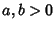anddefined by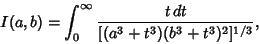(20)(21)

For iteration withandand(22)(23)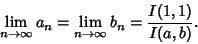(24)

Modular transformations are known when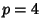and, but they do not give identities for(Borwein 1996).

References

Abramowitz, M. and Stegun, C. A. (Eds.). The Process of the Arithmetic-Geometric Mean.'' §17.6 in Handbook of Mathematical Functions with Formulas, Graphs, and Mathematical Tables, 9th printing. New York: Dover, pp. 571 ad 598-599, 1972.

Borwein, J. M. Problem 10281. A Cubic Relative of the AGM.'' Amer. Math. Monthly 103, 181-183, 1996.

Borwein, J. M. and Borwein, P. B. A Remarkable Cubic Iteration.'' In Computational Method & Function Theory: Proc. Conference Held in Valparaiso, Chile, March 13-18, 1989 (Ed. A. Dold, B. Eckmann, F. Takens, E. B Saff, S. Ruscheweyh, L. C. Salinas, L. C., and R. S. Varga). New York: Springer-Verlag, 1990.

Borwein, J. M. and Borwein, P. B. A Cubic Counterpart of Jacobi's Identity and the AGM.'' Trans. Amer. Math. Soc. 323, 691-701, 1991.

Press, W. H.; Flannery, B. P.; Teukolsky, S. A.; and Vetterling, W. T. Numerical Recipes in FORTRAN: The Art of Scientific Computing, 2nd ed. Cambridge, England: Cambridge University Press, pp. 906-907, 1992.